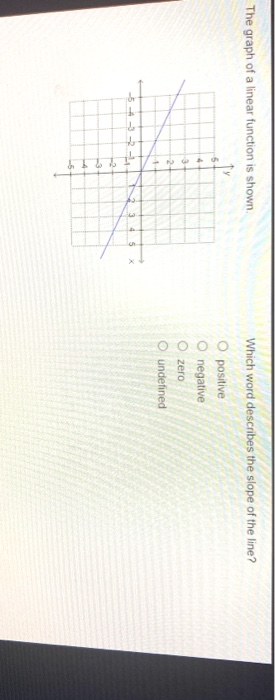# Which word describes the slope of the line?The graph of a linear function is shown Which word describes the slope of the line? positive negative Ozero undefined 2 5 2

Solution)
The question can be solved in two ways
1) by reasoning,
The line lies in the second and the 4th quadrant and thus the
angle ∅ it makes with the x axis lies in second quadrant where the
slope tan∅ value is NEGATIVE.
2) By writing equation
The line (as seen from the graph) passes through points
(0,0) & (2,-1)
Thus the slope of the line is
M= (-1-0)/(2-0)= -1/2= -0.5
Which is a negative value,
Thus the slope is Negative

Scroll to Top